# Introducing tidyterra

Easily work and ggplot SpatRasters

If you have been playing around with R for a while, probably you are familiarized with the `volcano` dataset:

``````
data("volcano")
image(volcano, col = terrain.colors(256, rev = TRUE))
``````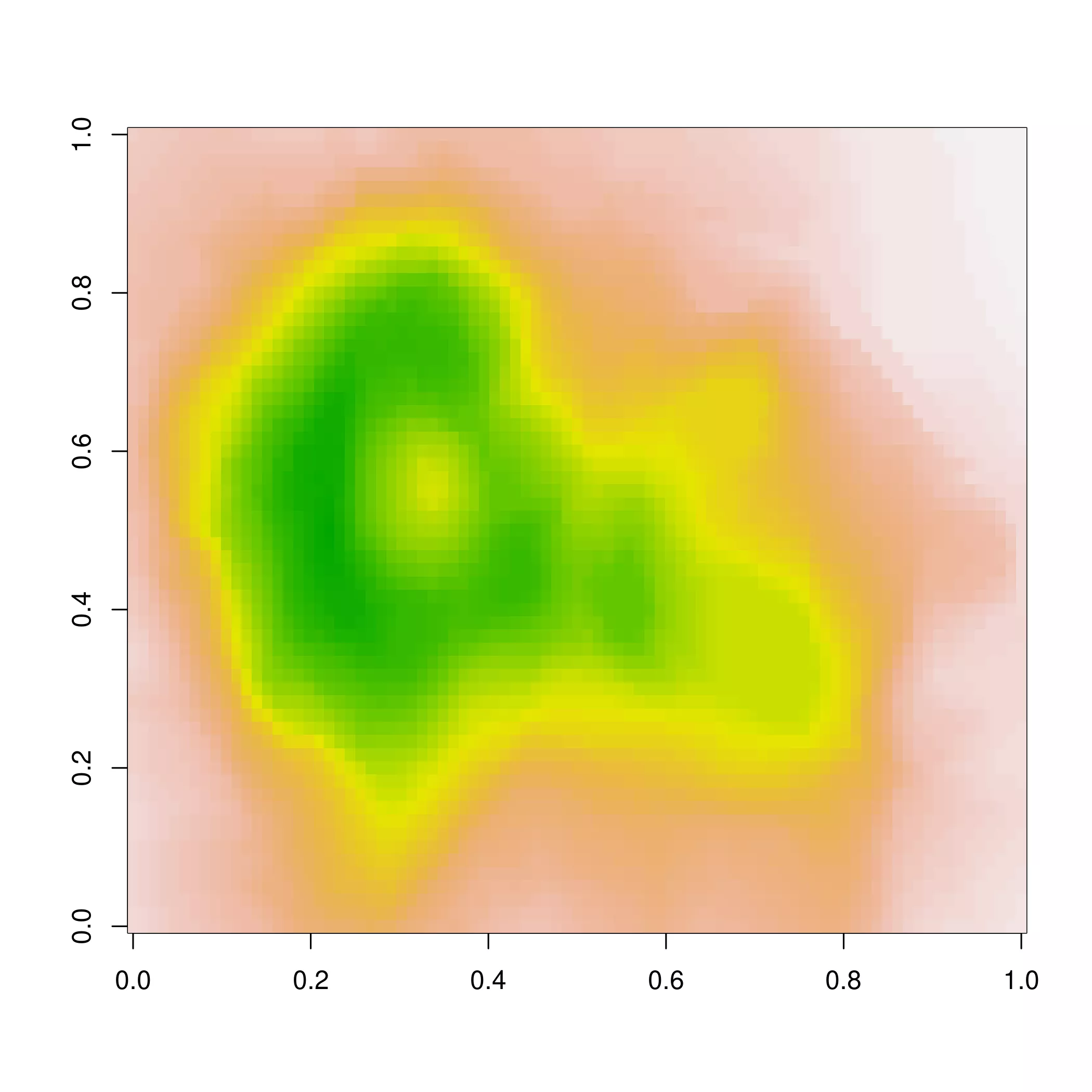This represents the topographic information of one of the volcanoes of Auckland (New Zealand), specifically Maungawhau / Mount Eden. But do you know that this map is flipped?

On this post I introduce the tidyterra package, recently added to CRAN and I would show you how to geotag the `volcano` dataset. We would produce also ggplot2 maps using the functions of `tidyterra`.

``````# Libraries
library(terra)
library(ggplot2)
library(tidyterra)
library(maptiles)
library(sf)
``````

## Wait, `volcano` is flipped?

Let’s check it out. Thanks to the package `maptiles` we can have a glimpse of the location of Maungawhau using map tiles (as Google Maps uses). We would use `tidyterra` for displaying the map tile:

``````
# location of Maungawhau

box <- c(
174.7611552780,
-36.8799200525,
174.7682380109,
-36.8719519780
)
class(box) <- "bbox"
box <- st_as_sfc(box)
st_crs(box) <- 4326

box <- box %>%
# To crs for NZGD49
st_transform(27200)

tile <- get_tiles(box, crop = TRUE, zoom = 16)

ggtile <- ggplot() +
geom_spatraster_rgb(data = tile)

ggtile
``````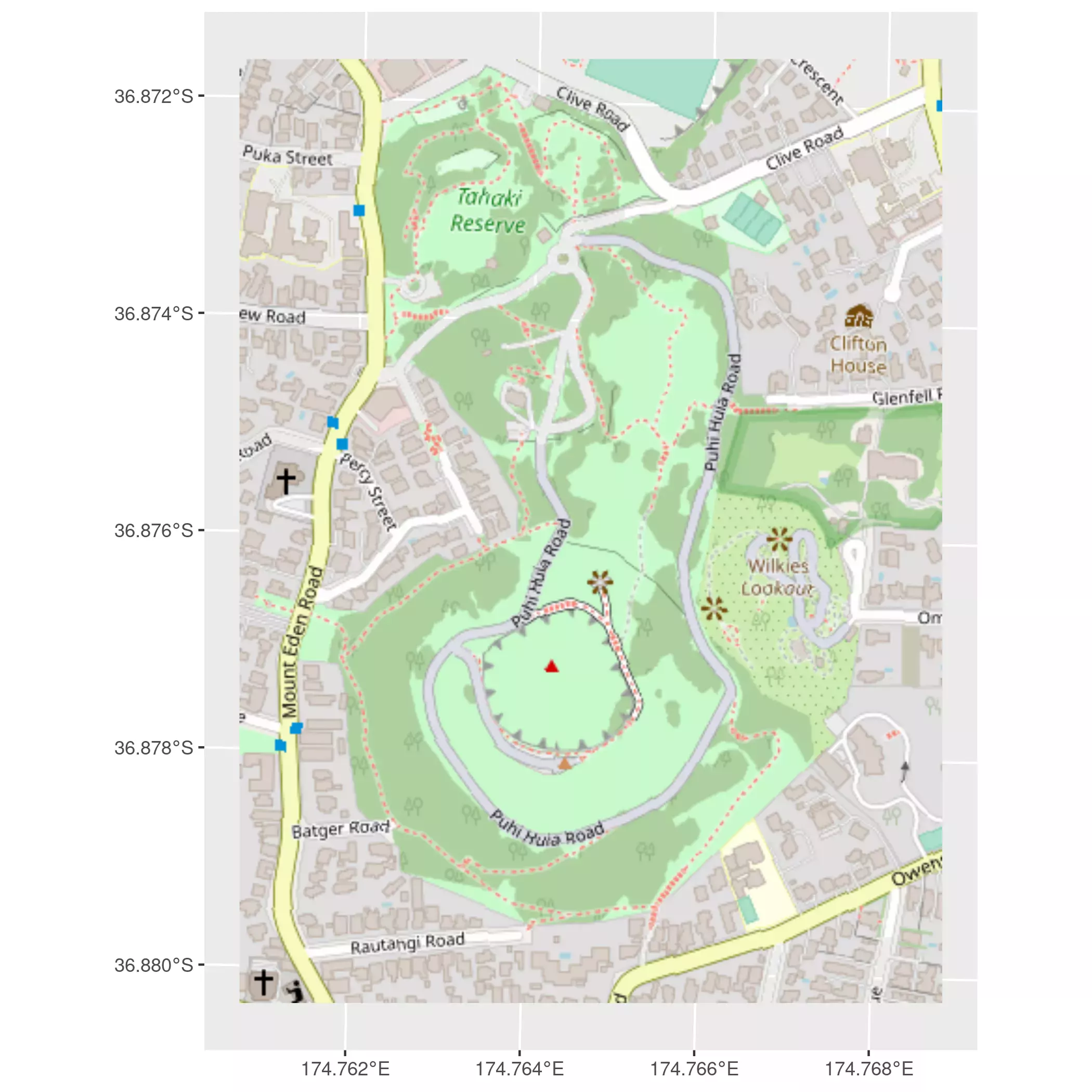So well, here you go. A neat and crisp RGB tile of Maungawhau. Now, the next question is, how to match the `volcano` dataset (a matrix) with this tile (a geo-tagged map tile)? Let’s check it out

## Working with SpatRasters

Thanks to the `terra` package we can start converting `volcano` into a SpatRaster:

``````
volcano_rast <- rast(volcano)

terra::plot(volcano_rast)
``````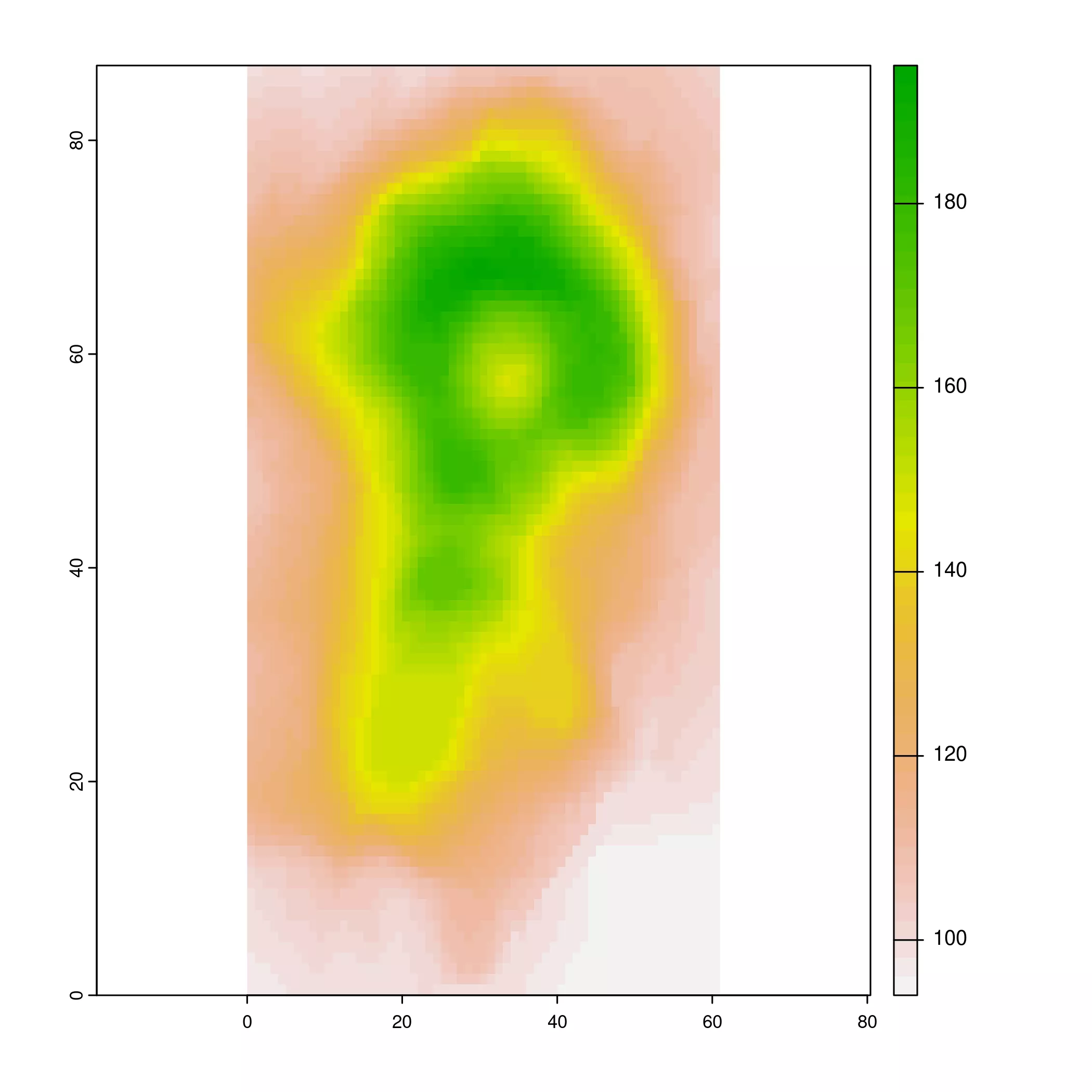``````
# Wait, it is flipped!
volcano_rast_ok <- rast(volcano[
seq(nrow(volcano), 1, -1),
seq(ncol(volcano), 1, -1)
])

# Much better!
terra::plot(volcano_rast_ok)
``````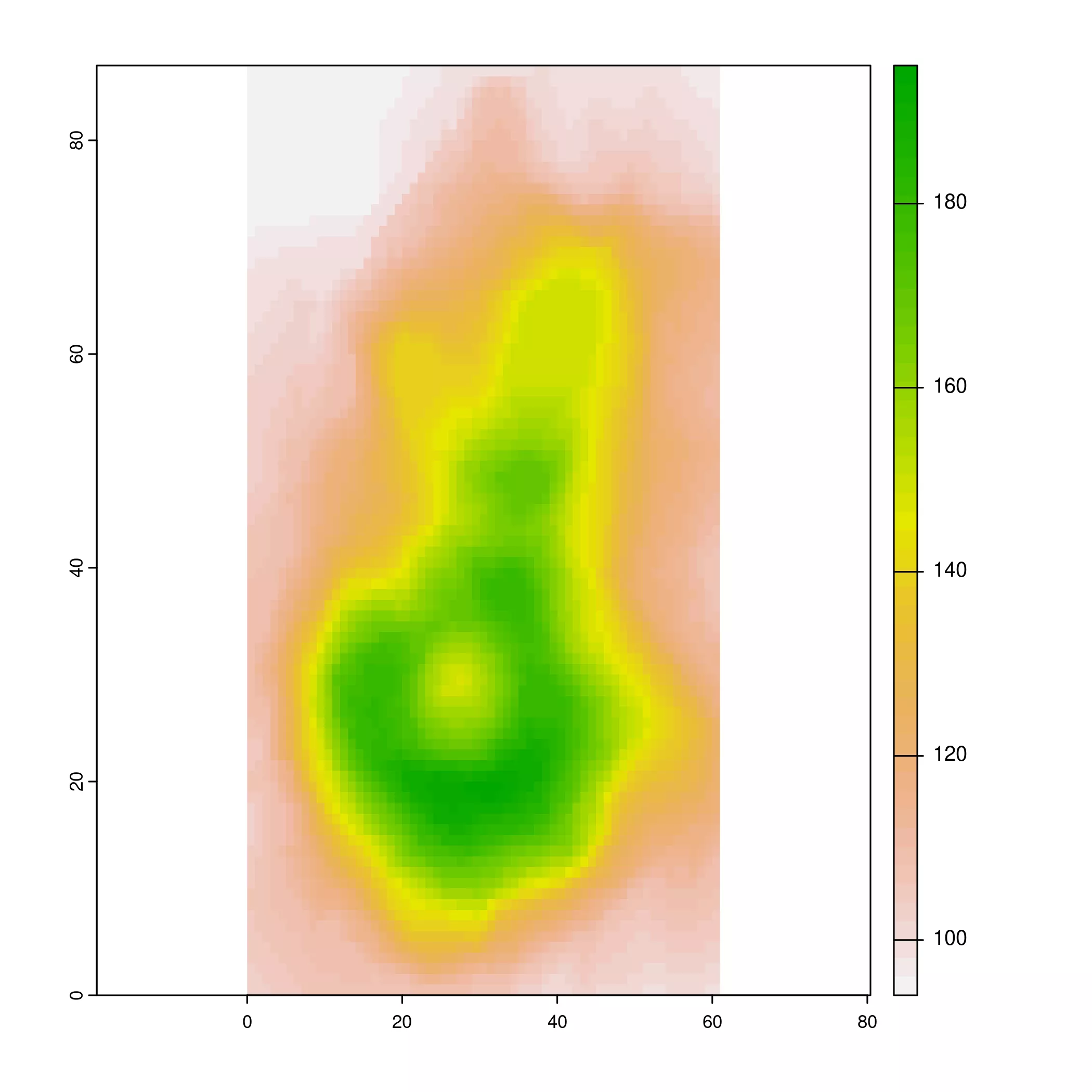``````
volcano_rast_ok
#> class       : SpatRaster
#> dimensions  : 87, 61, 1  (nrow, ncol, nlyr)
#> resolution  : 1, 1  (x, y)
#> extent      : 0, 61, 0, 87  (xmin, xmax, ymin, ymax)
#> coord. ref. :
#> source      : memory
#> name        : lyr.1
#> min value   :    94
#> max value   :   195
``````

Nice! Now we have a raster of `volcano`, but still without geotagged information. Thanks to this article of Tomislav Hengl (\@tom_hengl) we can check the basic geographic parameters of `volcano` (see Volcano Maungawhau), that are:

• CRS: EPSG:27200
• xllcorner: 2667400
• yllcorner: 6478700
• cellsize: 10 m
• ncols: 61
• nrows: 87

And we can translate that easily to an empty SpatRaster:

``````
# Extra length for proper handling extent
xrange <- range(seq(from = 2667400, length.out = 62, by = 10))
yrange <- range(seq(from = 6478700, length.out = 88, by = 10))

template <- rast(
crs = "EPSG:27200",
xmin = xrange,
xmax = xrange,
ymin = yrange,
ymax = yrange,
resolution = 10
)
template
#> class       : SpatRaster
#> dimensions  : 87, 61, 1  (nrow, ncol, nlyr)
#> resolution  : 10, 10  (x, y)
#> extent      : 2667400, 2668010, 6478700, 6479570  (xmin, xmax, ymin, ymax)
#> coord. ref. : NZGD49 / New Zealand Map Grid (EPSG:27200)
``````

So now we only need to transfer the values from `volcano_rast_ok` to our template:

``````
# Use tidyterra for pull the values of one raster
# and create a new layer

volcano2 <- template %>%
mutate(elevation = pull(volcano_rast_ok, lyr.1)) %>%
select(elevation)

volcano2
#> class       : SpatRaster
#> dimensions  : 87, 61, 1  (nrow, ncol, nlyr)
#> resolution  : 10, 10  (x, y)
#> extent      : 2667400, 2668010, 6478700, 6479570  (xmin, xmax, ymin, ymax)
#> coord. ref. : NZGD49 / New Zealand Map Grid (EPSG:27200)
#> source      : memory
#> name        : elevation
#> min value   :        94
#> max value   :       195

terra::plot(volcano2)
``````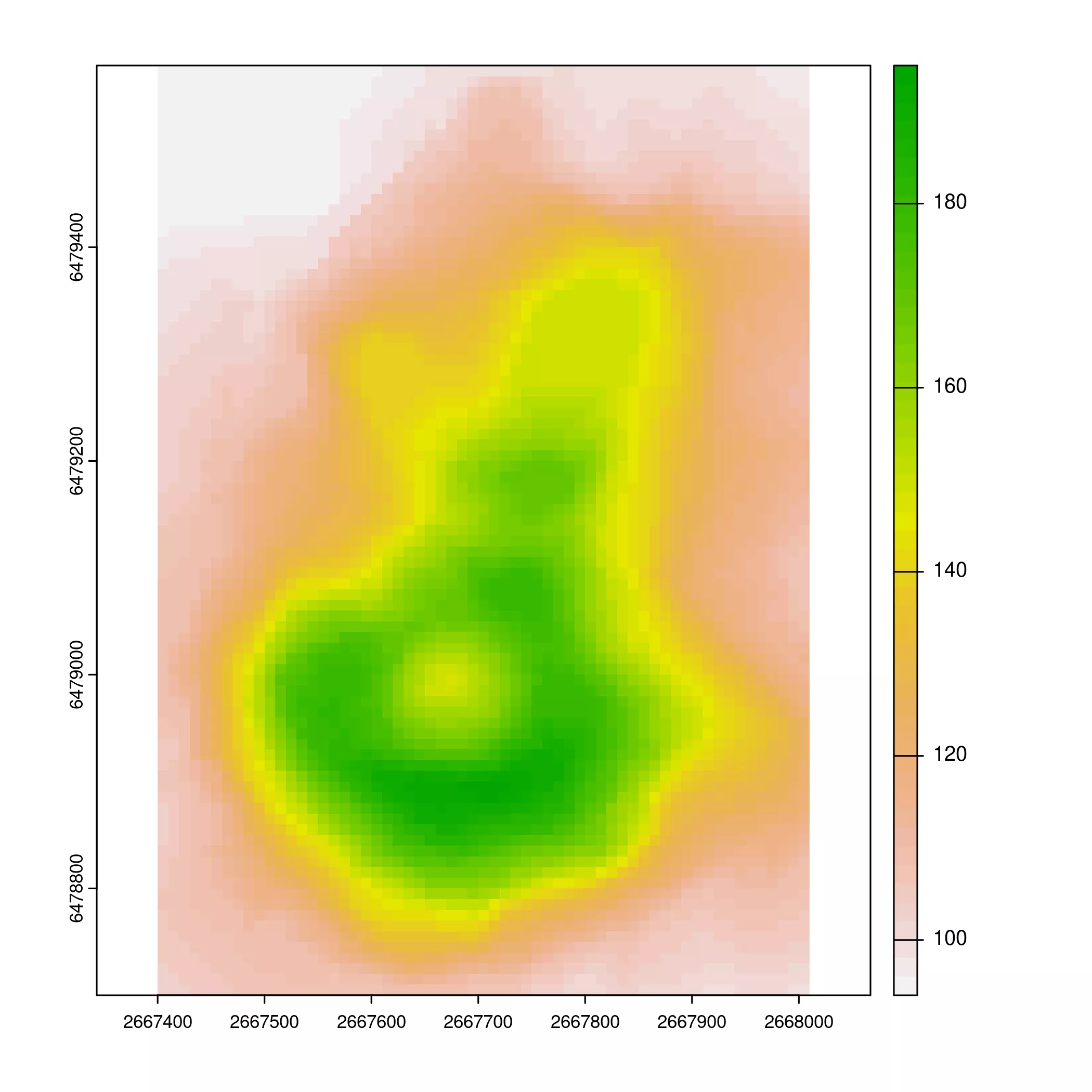``````
# And plot it
ggtile +
geom_spatraster(data = volcano2) +
scale_fill_terrain_c(alpha = 0.75)
``````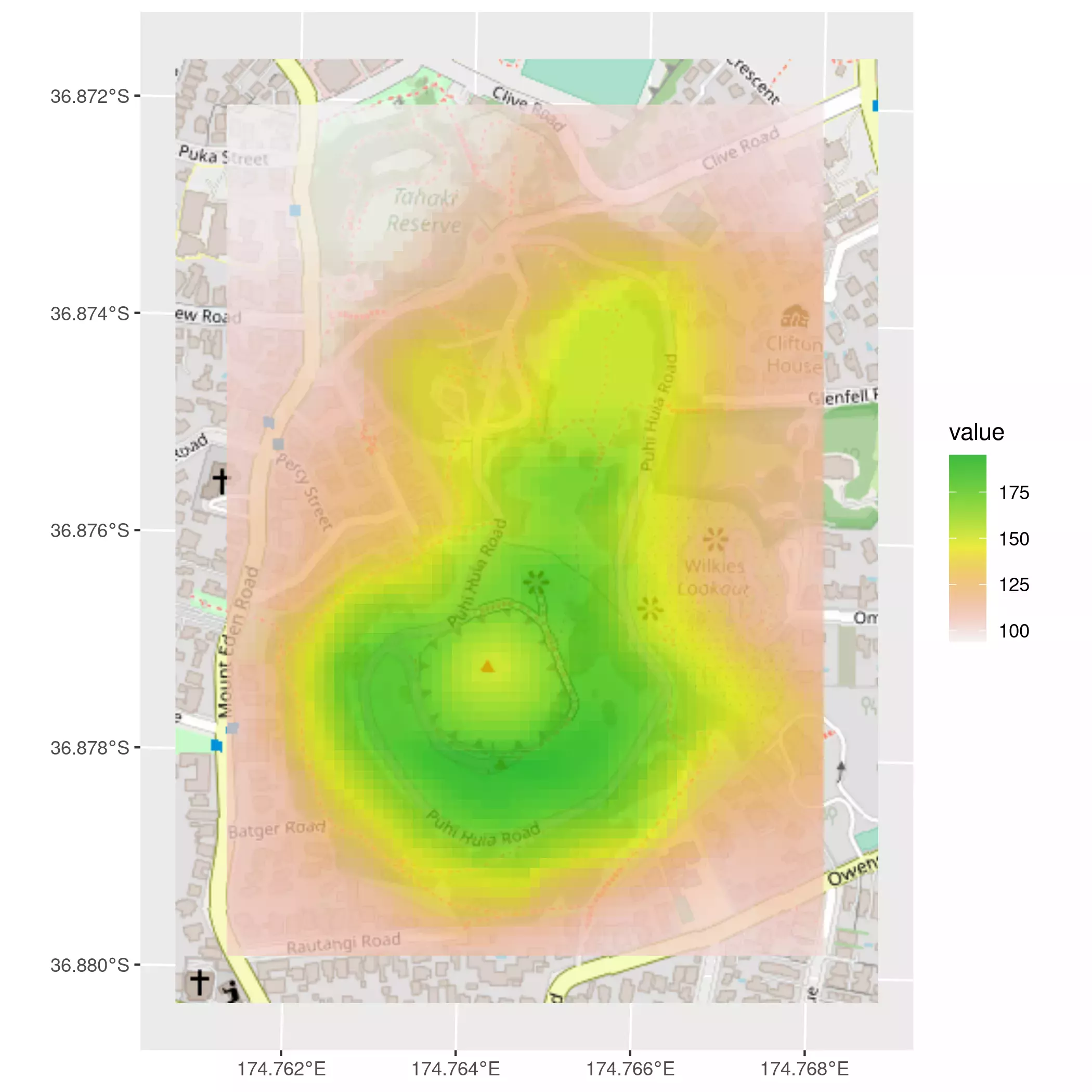## An Easter egg

The `volcano` dataset may not be completely up to date. As a compliment, `tidyterra` includes a `.tif` file with the same dimensions that our `volcano2` raster, but with the topographic values extracted from Auckland LiDAR 1m DEM (2013) and resampled to a resolution of 5x5 meters, for package size optimization. See here how to load it and check the plotting `tidyterra` possibilities:

``````

volcano2_easter <- rast(system.file("extdata/volcano2.tif",
package = "tidyterra"
))

volcano2_easter
#> class       : SpatRaster
#> dimensions  : 174, 122, 1  (nrow, ncol, nlyr)
#> resolution  : 5, 5  (x, y)
#> extent      : 1756969, 1757579, 5917003, 5917873  (xmin, xmax, ymin, ymax)
#> coord. ref. : NZGD2000 / New Zealand Transverse Mercator 2000 (EPSG:2193)
#> source      : volcano2.tif
#> name        : elevation
#> min value   :  76.26222
#> max value   :  195.5542
terra::plot(volcano2_easter)
``````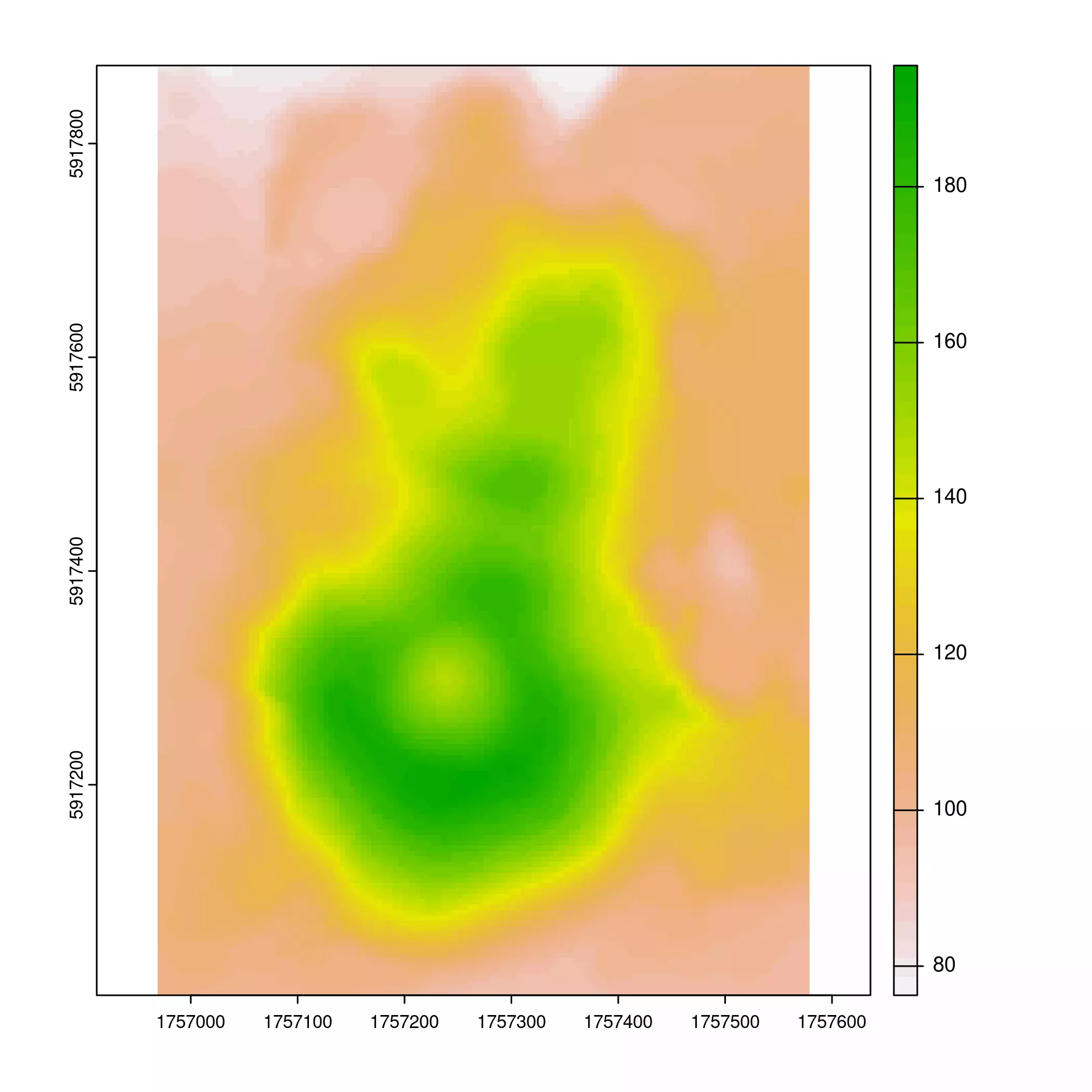``````

# Only altitudes of more than 130m

volcano_filter <- volcano2_easter %>%
filter(elevation > 130)

ggtile +
geom_spatraster(data = volcano_filter) +
scale_fill_viridis_c(na.value = NA, alpha = 0.7) +
labs(fill = "Elevation (m)")
``````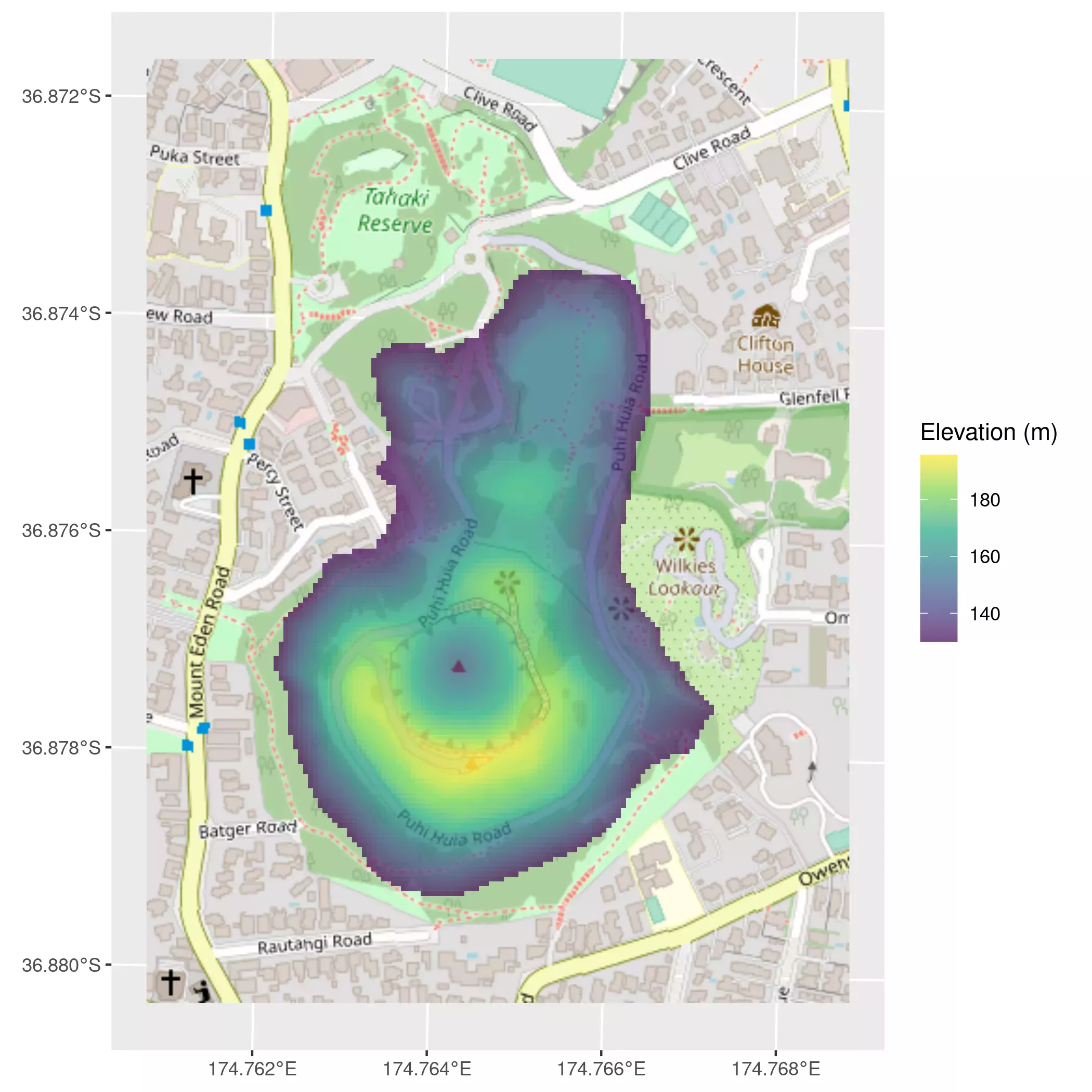``````

# Contour lines

ggtile +
geom_spatraster_contour(data = volcano2_easter, binwidth = 10)
``````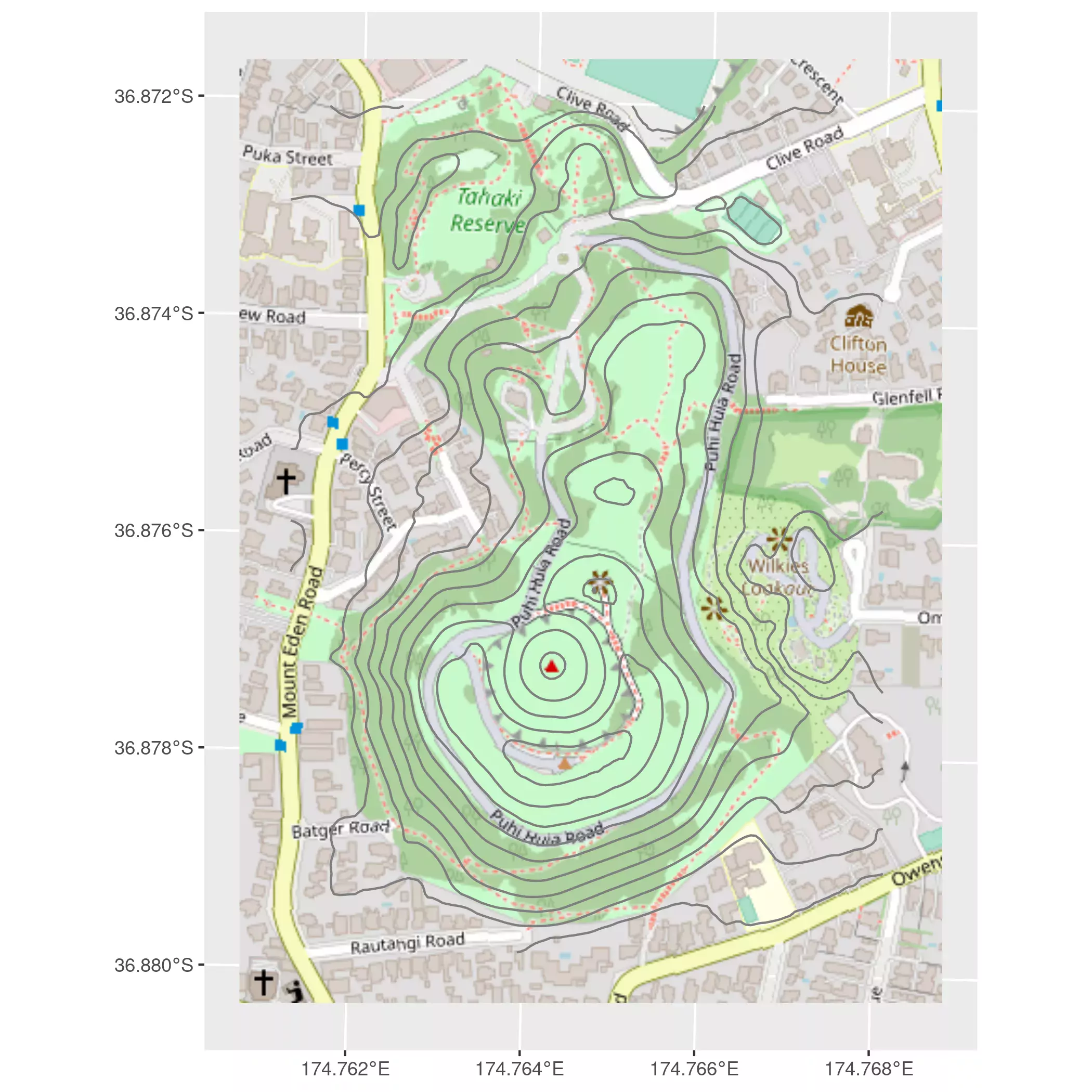``````

# Contour lines + contour polygons

ggtile +
geom_spatraster_contour_filled(
data = volcano2_easter,
breaks = seq(70, 210, 20),
alpha = 0.7
) +
geom_spatraster_contour(
data = volcano2_easter, binwidth = 2.5,
alpha = 0.7, size = .2, color = "grey10"
) +
coord_sf(expand = FALSE)
``````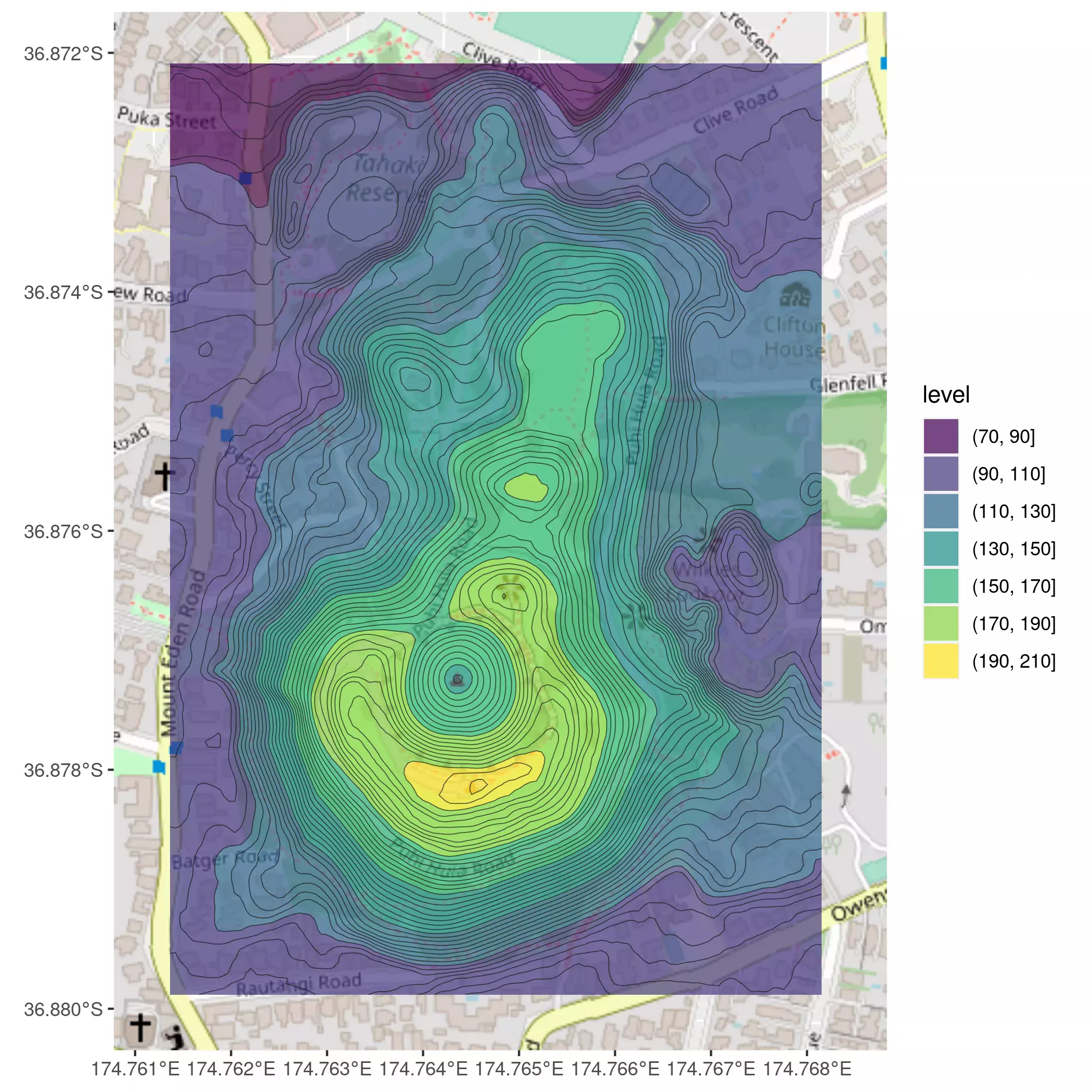Related